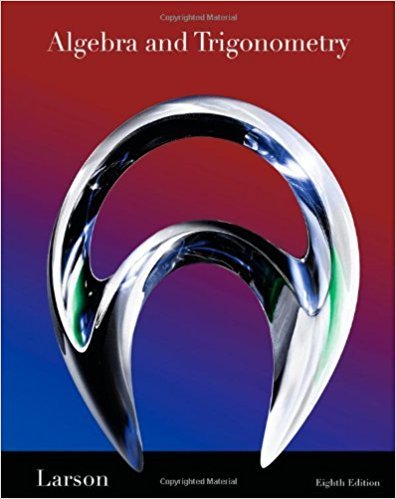×
×

# Solutions for Chapter 11.4: Mathematical Induction## Full solutions for Algebra and Trigonometry | 8th Edition

ISBN: 9781439048474Solutions for Chapter 11.4: Mathematical Induction

Solutions for Chapter 11.4
4 5 0 285 Reviews
27
0
##### ISBN: 9781439048474

This expansive textbook survival guide covers the following chapters and their solutions. Since 85 problems in chapter 11.4: Mathematical Induction have been answered, more than 48862 students have viewed full step-by-step solutions from this chapter. This textbook survival guide was created for the textbook: Algebra and Trigonometry, edition: 8. Algebra and Trigonometry was written by and is associated to the ISBN: 9781439048474. Chapter 11.4: Mathematical Induction includes 85 full step-by-step solutions.

Key Math Terms and definitions covered in this textbook
• Associative Law (AB)C = A(BC).

Parentheses can be removed to leave ABC.

• Cholesky factorization

A = CTC = (L.J]))(L.J]))T for positive definite A.

• Determinant IAI = det(A).

Defined by det I = 1, sign reversal for row exchange, and linearity in each row. Then IAI = 0 when A is singular. Also IABI = IAIIBI and

• Distributive Law

A(B + C) = AB + AC. Add then multiply, or mUltiply then add.

• Factorization

A = L U. If elimination takes A to U without row exchanges, then the lower triangular L with multipliers eij (and eii = 1) brings U back to A.

• Four Fundamental Subspaces C (A), N (A), C (AT), N (AT).

Use AT for complex A.

• Free columns of A.

Columns without pivots; these are combinations of earlier columns.

• Full column rank r = n.

Independent columns, N(A) = {O}, no free variables.

• Hermitian matrix A H = AT = A.

Complex analog a j i = aU of a symmetric matrix.

• Indefinite matrix.

A symmetric matrix with eigenvalues of both signs (+ and - ).

• Least squares solution X.

The vector x that minimizes the error lie 112 solves AT Ax = ATb. Then e = b - Ax is orthogonal to all columns of A.

• Left nullspace N (AT).

Nullspace of AT = "left nullspace" of A because y T A = OT.

• Markov matrix M.

All mij > 0 and each column sum is 1. Largest eigenvalue A = 1. If mij > 0, the columns of Mk approach the steady state eigenvector M s = s > O.

• Nullspace matrix N.

The columns of N are the n - r special solutions to As = O.

• Nullspace N (A)

= All solutions to Ax = O. Dimension n - r = (# columns) - rank.

• Rank r (A)

= number of pivots = dimension of column space = dimension of row space.

• Reflection matrix (Householder) Q = I -2uuT.

Unit vector u is reflected to Qu = -u. All x intheplanemirroruTx = o have Qx = x. Notice QT = Q-1 = Q.

• Simplex method for linear programming.

The minimum cost vector x * is found by moving from comer to lower cost comer along the edges of the feasible set (where the constraints Ax = b and x > 0 are satisfied). Minimum cost at a comer!

• Spanning set.

Combinations of VI, ... ,Vm fill the space. The columns of A span C (A)!

• Toeplitz matrix.

Constant down each diagonal = time-invariant (shift-invariant) filter.

×